# Civil Engineering - UPSC Civil Service Exam Questions

36.

A steel frame is shown in the given figure.
If joint O of the frame is rigid, the rotational stiffness of the frame at point O is given by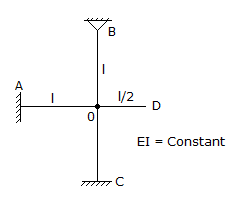A.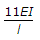B.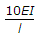C.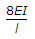D.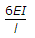Explanation:

No answer description available for this question. Let us discuss.

37.

A circle of radius 7 m has a standard error of 0.02 m on the radius. The standard error of its area is

 A. 0.04 m2 B. 0.14 m2 C. 0.28 m2 D. 0.88 m2

Explanation:

No answer description available for this question. Let us discuss.

38.

A circular shaft is subjected to a twisting moment T and bending moment M. The ratio of maximum bending stress to maximum shear stress is given by

 A.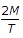B.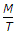C.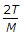D.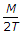Explanation:

No answer description available for this question. Let us discuss.

39.

By using sieve analysis, the particle size distribution curve has been plotted for a particular soil. The coefficient of curvature Cc is given by

 A.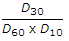B.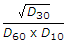C.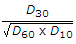D.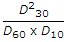Explanation:

No answer description available for this question. Let us discuss.

40.

Consider the following properties :
1. The sum of the three sides is always greater than the circumference of the great circle.
2. The sum of the three angles is less than six right angles and greater than two right angles.
3. The sum of the sides is greawter than the third side.
4. The smaller angle is opposite the smaller side and vice versa.
The propoerties of spherical triangles would include

 A. 1, 2 and 3 B. 2, 3 and 4 C. 1, 2 and 4 D. 1, 3 and 4